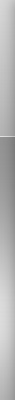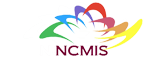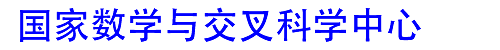网站地图 | 联系我们 | English | 意见反馈 | 主任信箱
 首页中心概况新闻动态科研进展交流合作人才培养研究队伍人才招聘政策规章数学交叉科学传播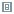科研进展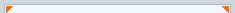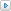科研成果研究专题获奖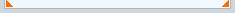现在位置：首页 > 科研进展 > 科研成果
 维度发散时间序列的模型平均预测 【大 中 小】【打印】【关闭】 2021-9-8 维度发散时间序列的模型平均预测（张新雨）In time series analysis, the autoregressive model serves as a primary tool. Early relevant literature, such as Hannan (1969), Box and Jenkins (1970), Parzen (1974), Anderson (1977) and Hamilton (1994), often focuses on the inference of ARMA(p, q) with p and q known. However, in practice, the structure of the true model is unknown and a common approach is to approximate the true model by a model including many parameters; see, for example, Ing and Wei (2005) and Bickel and Gel (2011). Especially, under the assumption that data are generated from an AR(∞) model, Berk (1974), Shibata (1977) and Bhansali (1978) approximated the true model by fitting an autoregressive model of order k. Subsequently, an inevitable issue is how to select the order k. There exists rich literature on methods of selecting model order for the two different types of prediction cases, i.e., the independent-realization (IR) prediction and the same-realization (SR) prediction. With the IR prediction, we mean that the process to be predicted is independent of but has the same probability structure as the series used to estimate the model. With the SR prediction, we mean that the process to be predicted utilizes the same series as that used to estimate the model. It is clear that the SR prediction is just the common prediction case in practice. On the other hand, the IR assumption is often used for theoretical analysis on order selection in literature(Akaike, 1970; Shibata, 1980; Bhansali, 1996). In fact, for the IR case, because the variable to be predicted is independent of the regression coefficient estimator, the decomposition of mean squared prediction error (MSPE) and the derivations of theoretical properties can be simplified. However, the IR setting is an idealized case and rarely used in practice. A possible application of the IR assumption is when there are only a few observations of the interested series, in which case the estimation is infeasible or very unreliable and we can use the model estimated by the series with similar features. In an IR prediction setting, Shibata (1980) showed that the three criteria of order selection, the final prediction error (FPE) (Akaike, 1970), AIC (Akaike, 1974) and his proposed Sn(k), are asymptotically efficient in the sense of minimizing MSPE. This result was extended to the multistep prediction case by Bhansali (1996). In an SR prediction setting, Ing and Wei (2003) obtained an asymptotically equivalent expression for MSPE. Furthermore, Ing and Wei (2005), based on Ing and Wei (2003), provided the first rigorous verification that AIC and Cp (Mallows, 1973) are asymptotically efficient in the sense of minimizing MSPE. Ing (2007) explored the predictive power of the corrected Rissanen’s accumulated prediction error criterion in an AR(∞) model. Ing et al. (2012) further demonstrated that AIC and its variants are also asymptotically efficient in the sense of achieving the lowest MSPE for the SR prediction in integrated AR(∞) processes. See Ng (2013) for a useful review on variable selection in predictive regressions.However, selecting a single model may lose the information contained in other models. Also, the model selected on the basis of finite samples may be poor. An approach to overcoming the shortcomings is model averaging, which, serving as a smoothed extension of model selection from the aspects of estimation and forecast, has received considerable attention in recent years. Model averaging combines all of candidate models by certain weights, and so it avoids the risk of ‘‘putting all eggs in one basket’’. The key problem with the model averaging approach is the choice of weights.In this paper, a generalized Mallows model averaging (GMMA) criterion for choosing weights is developed in the context of an infinite order autoregressive (AR(∞)) process. The GMMA method adapts to the circumstances in which the dimensions of candidate models can be large and increase with the sample size. The GMMA method is shown to be asymptotically optimal in the sense of achieving the lowest out-of-sample mean squared prediction error (MSPE) for both the independent-realization and the same-realization predictions, which, as a byproduct, solves a conjecture put forward by Hansen (2008) that the well-known Mallows model averaging criterion from Hansen (2007) is asymptotically optimal for predicting the future of a time series. The rate of the GMMA-based weight estimator tending to the optimal weight vector minimizing the independent-realization MSPE is derived as well. Both simulation experiment and real data analysis illustrate the merits of the GMMA method in the prediction of an AR(∞) process.Publication:- Journal of Econometrics, 223, 1, 190-221 (2021).Authors:- Jun Liao (Renmin University of China)- Guohua Zou (Capital Normal University)- Yan Gao (Minzu University of China)- Xinyu Zhang (Academy of Mathematics and Systems Science, Chinese Academy of Sciences)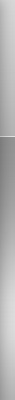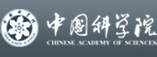欢迎访问国家数学与交叉科学中心　 地址：北京海淀区中关村东路55号　邮编：100190　电话: 86-10-62613242 Fax: 86-10-62616840 邮箱: ncmis@amss.ac.cn